# Calculator skills

Here you will learn about calculator skills, including the buttons that help us navigate the calculator display with ease and how to compute basic calculations to demonstrate calculator proficiency.

Students will first learn about calculator skills as part of number and operations in elementary school and the number system in middle school.

## What are calculator skills?

To do this, take a look at the digital calculator below.

The digits 0-9, the decimal point, and the four main operations are in the middle of the calculator.

Let’s look at some examples to explore the different calculator skills.

### Simple calculations

For example,

To solve 56.1 + 3…

Commands:

• Type 56.1
• Press +
• Type 3

This calculator does not separate larger numbers with commas. For example, type 34,000 into the calculator as 34000.

At any point if you make a mistake, use the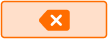button to delete and try again.

Once you have completed a calculation, you can keep it by pressing the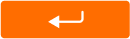key or the ‘enter’ key on your keyboard. This moves the equation up, so that you can still see it even after you start a new equation.

Or, if you are done with the calculation, you can press ‘clear all.’

### Calculations with fractions

To create a fraction use the \, \cfrac{a}{b} \, button and then enter the numerator and the denominator.

For example,

To solve 2\cfrac{1}{2} \times \cfrac{2}{3}…

Commands:

• Type 2
• Press \, \cfrac{a}{b} \, and then enter 1 as the numerator and 2 as the denominator
• Click to the right of the fraction
• Press \times
• Press \, \cfrac{a}{b} \, and then enter 2 as the numerator and 3 as the denominator

This calculator uses a dot symbol for multiplication. Multiplication can be shown with a variety of symbols: \, \times \; \cdot \; * \;\, ( \, )

The answer is shown as a decimal. Click the buttonto show the answer as an improper fraction.

### Multi step calculations

If you want to solve a multi-step calculation, there are two options.

First, you can use the ‘ans’ key and enter each step separately.

For example,

To solve 5.6-3.4 and then divide the answer by 2…

• Type 5.6
• Press -
• Type 3.4
• Press enter
• Press ans
• Press \div
• Type 2

This calculator uses the fraction bar symbol to represent division.

Or, you can use parentheses as a part of the order of operations.

• Press (
• Type 5.6
• Press -
• Type 3.4
• Press )
• Press \div
• Type 2

### Exponents

To solve with exponents, use the a^2 button or a^b. The a^2 squares any number by using the exponent 2.

The a^b button lets you enter any base and exponent.

For example,

To solve 6^4…

• Type 6
• Press a^b
• Enter 4 as the exponent

To find the percent of a number, use the \% button.

For example,

To solve 40\% of 50…

• Type 40
• Press \%
• Type 50

### Absolute value

To find the absolute value, use the |a| button.

For example,

To solve |−1| + |9|…

• Press |a|
• Type -1
• Click to the right
• Press +
• Press |a|
• Type 9

### What are calculator skills?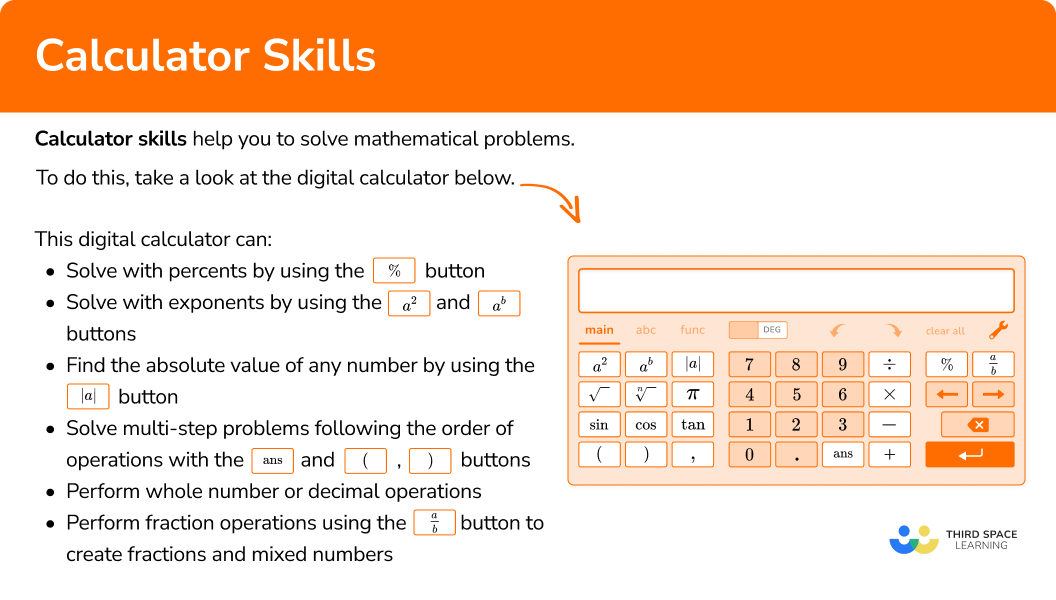## Common Core State Standards

How does this relate to 5th grade math and 6th grade math?

• Grade 5 – Operations and Algebraic Thinking (5.OA.A.1)
Use parentheses, brackets, or braces in numerical expressions, and evaluate expressions with these symbols.

• Grade 6 – Expressions and Equations (6.EE.A.1)
Write and evaluate numerical expressions involving whole-number exponents.

• Grade 6 – Ratios and Proportions (6.RP.A.3c)
Find a percent of a quantity as a rate per 100 (for example, 30\% of a quantity means \cfrac{30}{100} times the quantity); solve problems involving finding the whole, given a part and the percent.

## How to use calculator skills

In order to carry out calculations on a calculator:

1. Decide which calculator buttons to use.
2. Enter the calculation into the calculator.
3. Check the calculator display matches the question exactly.

## Calculator skills examples

### Example 1: whole number calculation on a calculator

Solve 34 + 459.

1. Decide which calculator buttons to use.

Use the middle buttons (the digits and the basic operations) to solve this equation.

2Enter the calculation into the calculator.

• Type 34
• Press +
• Type 459

3Check the calculator display matches the question exactly.

34 + 459 = 493

### Example 2: fraction calculation on a calculator

Solve \, \cfrac{3}{4} \times \cfrac{1}{5} \, . Give your answer as a mixed number.

Use the middle buttons (the digits and the basic operations) and the fraction button to solve this equation.

• Press \, \cfrac{a}{b} \, and then enter 3 as the numerator and 4 as the denominator
• Click to the right of the fraction
• Press \times
• Press \, \cfrac{a}{b} \, and then enter 1 as the numerator and 5 as the denominator

To convert 0.15 to a fraction, click thebutton.

\cfrac{3}{4} \times \cfrac{1}{5}=\cfrac{3}{20}

### Example 3: decimal calculation with order of operations on a calculator

Solve (10.1-2.4) \div 7+1.

Use the middle buttons (the digits and the basic operations) and the parentheses buttons to solve this equation.

• Press (
• Type 10.1
• Press -
• Type 2.4
• Press )
• Press \div
• Type 7
• Click to the right
• Press +
• Type 1

If you do not ‘click to the right’ after typing 7, the calculator will show this (below). Notice how it changes the order of operations and the answer. This is why it is important to check that the display matches the equation exactly.

(10.1-2.4) \div 7+1=2.1

### Example 4: exponent calculation on a calculator

Solve 7^3+4.

Use the middle buttons (the digits and the basic operations) and the a^b button to solve this equation.

• Type 7
• Press a^b
• Type 3
• Click to the right
• Press +
• Type 4

7^3+4=347

### Example 5: percent calculation on a calculator

What is 15\% of 360?

Use the middle buttons (the digits) and the \% button to solve this equation.

• Type 15
• Press \%
• Type 360

15\% of 360 is 54.

### Example 6: absolute value calculation on a calculator

Solve |−11|-|−3|.

Use the middle buttons (the digits and the basic operations) and the |a| button to solve this equation.

• Press |a|
• Type -11
• Click to the right
• Press -
• Press |a|
• Type -3

|−11|-|−3| = 8

### Teaching tips for calculator tips

• Do not introduce a new calculator to students while they are also learning a new math topic. Start with just calculator practice for a skill students have already mastered. This will allow students to focus on just learning how to use the calculator.

• When using worksheets, instead of providing an answer key, let students solve on their own and then check their work with a calculator. This helps them to continue to become more proficient with a calculator.

• Using the calculator takes the burden of calculating off a student and allows them to focus on the problem solving part of a skill. For example, when solving multi-step word problems with a calculator, students can focus on figuring out the operations and the order to perform them, instead of performing the calculations.

• A calculator is a great tool to use in the classroom to observe patterns. For example, when students are learning about Powers of 10, instead of telling them the rule, ask students to calculate 8.9 times 10, then 100, then 1,000 then 10,000, with the purpose of observing and discussing how the product changes each time.

### Easy mistakes to make

Pay close attention to how you should give your answer — what form it should be in and whether it should be rounded.
For example,
Solving 3 \cfrac{1}{2}+\cfrac{7}{8} \, with a calculator will show the answer as 4.375.
If the question asks you to provide the answer as a mixed number (or the answer choices are all mixed numbers), you will need to convert the calculator answer in order to finish solving.

• Misentering a multi-step equation
If you need to do more than one step, make sure you enter each step one at a time and use the ‘ans’ button OR enter the equation into the calculator following the order of operations.
For example,
To calculate \cfrac{1}{2}+3, you need to click to the right of the fraction before pressing + and typing 3.
Otherwise, it will go into the calculator as \cfrac{1}{2 \, + \, 3} \, , which changes the order of operations and the answer.

• Not using mental estimation to catch mistakes
Calculators are useful tools, but it is important not to become so dependent on them that you stop considering whether or not the answer makes sense.
For example,
Solve 2.3 \times 4.5 and consider if the answer makes sense. If the calculator shows 103.5 as the answer, that should be a clue that something went wrong.
Since 2.3 rounds to 2 and 4.5 rounds to 5, the answer should be close to 2 \times 5=10 . and 103.5 is too big. This tells you to re-enter the calculation and try again.

### Practice calculator skills questions

1. Use your calculator to solve 5,679,233 + 34,501.

5,713,734602,4245,644,7325,682,684• Type 5679233
• Press +
• Type 34501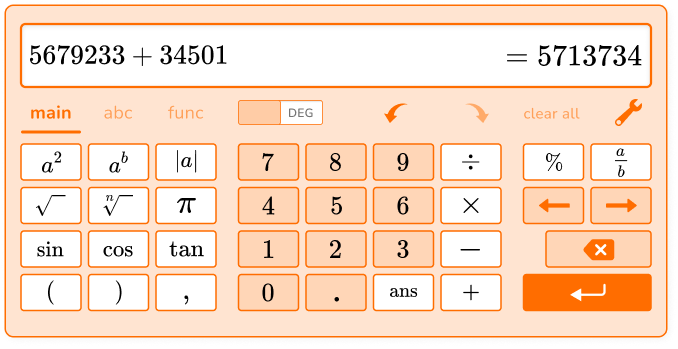Add a comma every 3 numbers.

5,679,233 + 34,501 = 5,713,734

2. Use your calculator to solve 7.6 \times (14.5-3.3).

135.28106.985.121,098.7• Type 7.6
• Press (
• Type 14.5
• Press
• Type 3.3
• Press )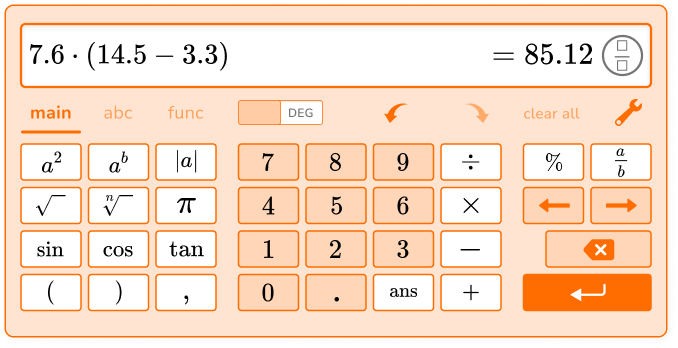7.6 \times (14.5-3.3)=85.12

3. Use your calculator to solve 3 \cfrac{1}{2} \div \cfrac{1}{4}.

\cfrac{7}{8}614\cfrac{1}{14}• Type 3
• Press \cfrac{a}{b} and then enter 1 as the numerator and 2 as the denominator
• Click to the right
• Press \div
• Press \cfrac{a}{b} and then enter 1 as the numerator and 4 as the denominator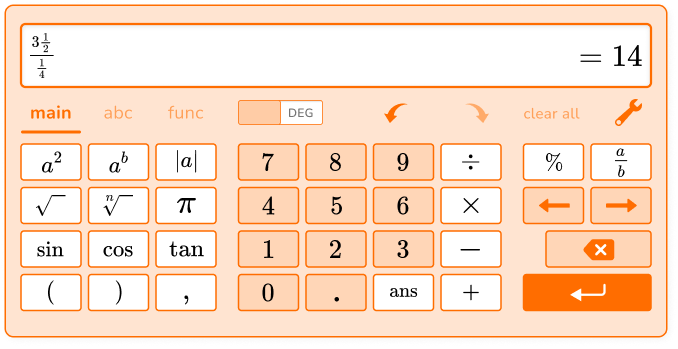3 \cfrac{1}{2} \div \cfrac{1}{4}=14

4. Use your calculator to solve 108-2^6.

64449612• Type 108
• Press
• Type 2
• Press a^b
• Type 6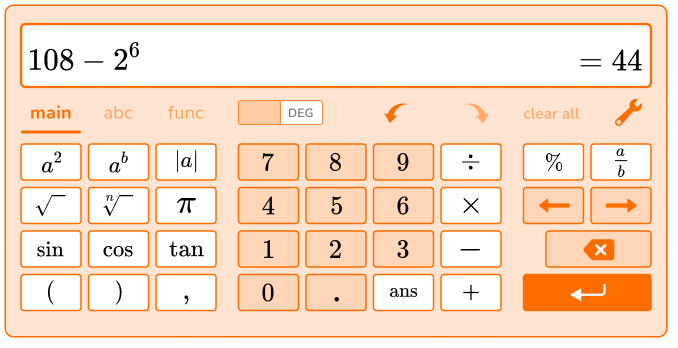108-2^6=44

5. Use your calculator to solve 90\% of 520.

57246845052• Type 90
• Press \%
• Type 520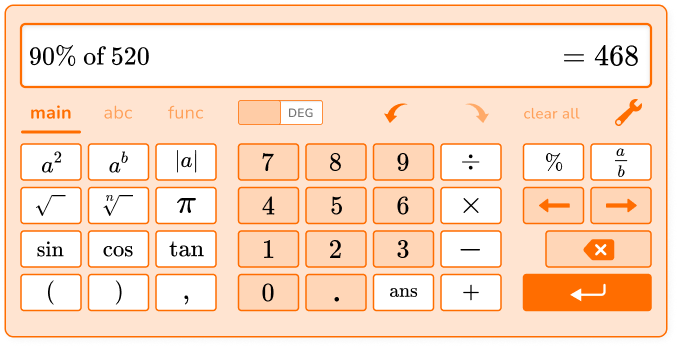90\% of 520 is 468.

6. Use your calculator to solve 3 \times |6+8|.

263014442• Type 3
• Press \times
• Click to the right
• Press |a|
• Type 6
• Press +
• Type 8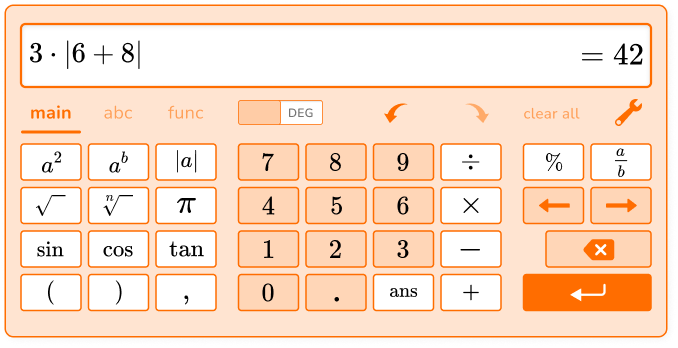3 \times |6+8|=42

## Calculator skills FAQs

What is the difference between a basic calculator, a scientific calculator and a graphing calculator?

A basic calculator includes digits 0-9 and buttons for the operations addition, subtraction, multiplication and division. A scientific calculator expands on a basic calculator and has buttons that support calculations for topics covered in middle school, high school and beyond. A graphing calculator has capabilities to solve and graph equations with variables, among other advanced functions.

Where is the negative symbol on a calculator?

Some calculators may have a separate button to create a negative value, but often the subtraction symbol is used for negatives as well.

Where to find calculators?

While you can purchase a handheld calculator, there are many other ways to access a calculator. Most smartphones include apps that have at least a basic calculator. There are also many free resources available online, many which provide tutorials on how to use them.

## Still stuck?

At Third Space Learning, we specialize in helping teachers and school leaders to provide personalized math support for more of their students through high-quality, online one-on-one math tutoring delivered by subject experts.

Each week, our tutors support thousands of students who are at risk of not meeting their grade-level expectations, and help accelerate their progress and boost their confidence.